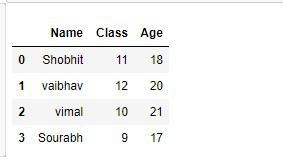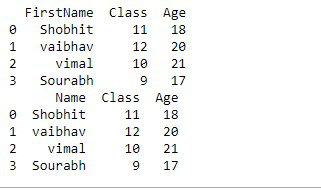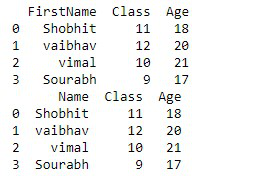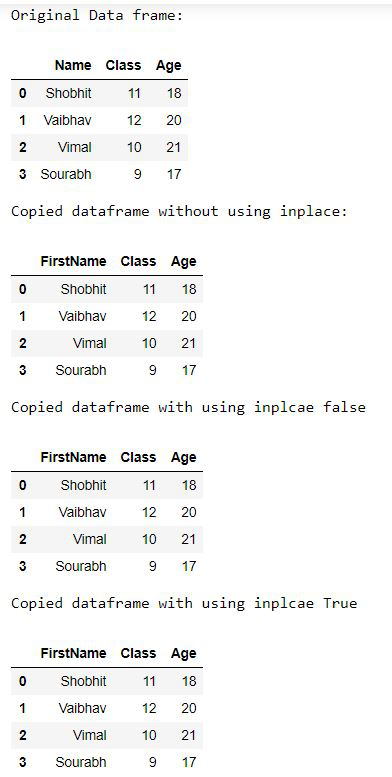Related Articles

# What does inplace mean in Pandas?

• Difficulty Level : Easy
• Last Updated : 11 Dec, 2020

In this article, we will see Inplace in pandas. Inplace is an argument used in different functions. Some functions in which inplace is used as an attributes like, set_index(), dropna(), fillna(), reset_index(), drop(), replace() and many more. The default value of this attribute is False and it returns the copy of the object.

Here we are using fillna() methods.

Syntax: dataframe.fillna(dataframe.mean(), inplcae = False)

Let’s understand this method with step-wise implementation:

Step 1. First, we import all the required libraries.

## Python3

 `# import required module``import` `pandas as pd`

Step 2.Creating dataframe.

## Python3

 `# creating dataframe``dataframe ``=` `pd.DataFrame({``'Name'``:[``'Shobhit'``,``'vaibhav'``,``                                ``'vimal'``,``'Sourabh'``],``                         ` `                        ``'Class'``:[``11``,``12``,``10``,``9``],``                        ``'Age'``:[``18``,``20``,``21``,``17``]})`` ` `# Checking created dataframe``display(dataframe)`

Output :Step 3.To see the inplace use we are going to use the rename function where we are renaming “Name” Column to “FirstName”.

In this step, We will not use inplace in our code.

## Python3

 `# without using inplace renaming the column``new_data ``=` `dataframe.rename(columns ``=` `{``'Name'``:``'FirstName'``})`` ` `# check new_data``display(new_data)`

Output :We can clearly see that there are no changes in the original dataframe. Through this, we conclude that the default value of inplace is False.

Now in this step, we will use inplace with False value.

## Python3

 `# putting inplace=False``new_data_2 ``=` `dataframe.rename(columns ``=` `{``'Name'``:``'FirstName'``},``                            ``inplace ``=` `False``)`` ` `#check new_data_2``display(new_data_2)`

Output :Again we can clearly see that there are no changes in the original dataset.

At last, we are putting inplace value equal to True.

## Python3

 `# Putting Inplace=True``dataframe.rename(columns ``=` `{``'Name'``:``'FirstName'``},``                 ``inplace ``=` `True``)``  ` `# check whether dataframe is modidfied or not``print``(dataframe)`

Output :Finally, we can see that the original dataframe columns have been modified from “Name” to “FirstName”.

Below is the complete program based on the above approach :

## Python3

 `# importing pandas``import` `pandas as pd`` ` `# creating dataframe``dataframe``=``pd.DataFrame({``'Name'``:[``'Shobhit'``,``'Vaibhav'``,``                                ``'Vimal'``,``'Sourabh'``],``                         ` `                        ``'Class'``:[``11``,``12``,``10``,``9``],``                        ``'Age'``:[``18``,``20``,``21``,``17``]})`` ` `# Checking created dataframe``# copied dataframe``display(dataframe)`` ` `# without using inplace renaming the column``new_data ``=` `dataframe.rename(columns ``=` `{``'Name'``:``'FirstName'``})`` ` `# Copied dataframe``display(new_data)  `` ` `# checking whether dataframe is modified or not``# Original dataframe``display(dataframe)`` ` `# putting inplace=False``new_data_2 ``=` `dataframe.rename(columns ``=` `{``'Name'``:``'FirstName'``},``                              ``inplace ``=` `False``)`` ` `# Copied dataframe``display(new_data_2)`` ` `# checking whether dataframe is modified or not``# Original dataframe``display(dataframe)`` ` `# Putting Inplace=True``dataframe.rename(columns ``=` `{``'Name'``:``'FirstName'``},``                 ``inplace ``=` `True``)`` ` `# checking whether dataframe is modified or not``# Original dataframe``display(dataframe)`

Output :Attention geek! Strengthen your foundations with the Python Programming Foundation Course and learn the basics.

To begin with, your interview preparations Enhance your Data Structures concepts with the Python DS Course. And to begin with your Machine Learning Journey, join the Machine Learning – Basic Level Course

My Personal Notes arrow_drop_up